# Fence and scale

The garden with 80 m of fencing is draw on plan as a square with a side length of 4 cm. At what scale is this plan made?

Result

M = (Correct answer is: 1/500)#### Solution: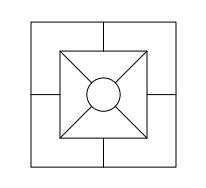We would be pleased if you find an error in the word problem, spelling mistakes, or inaccuracies and send it to us. Thank you!#### You need to know the following knowledge to solve this word math problem:

We encourage you to watch this tutorial video on this math problem:

## Next similar math problems:

• GardenArea of a square garden is 6/4 of triangle garden with sides 56 m, 35 m, and 35 m. How many meters of fencing need to fence a square garden?
• ScaleSwimming pool is long 110 m and 30 m wide. The plan of the city is shown as a rectangle with area 8.25 cm2. What scale is the city plan?
• GardenRectangular garden 31 meters 40 centimeters long and 20m30cm wide adjacent with the shorter side with another (fenced) garden and on one longest side is 1 meter wide gate. How many meters of fence is needed to buy? (Estimate, calculate, verify a test calc
• The sidesThe sides of a rectangle are in a ratio of 2:3, and its perimeter is 1 1/4 inches. What are the lengths of its side? Draw it.
• Map scaleOn a 1:1000 scale map is a rectangular land of 4.2 cm and 5.8 cm. What is the area of this land in square meters?
• Plan of the villagePlan of the municipality in 1:1000 scale has plotted garden with dimensions 25 mm and 28 mm. Determine the area of gardens in ares in reality.
• Square garden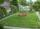On the plan with a scale of 1:1500 is drawn as a square garden with area 81 cm2. How many meters is long garden fence? Determine the actual acreage gardens.
• Squares ratioThe first square has a side length of a = 6 cm. The second square has a circumference of 6 dm. Calculate the proportions of the perimeters and the proportions of the contents of these squares? (Write the ratio in the basic form). (Perimeter = 4 * a, conte
• PrismRight angle prism, whose base is right triangle with leg a = 3 cm and hypotenuse c = 13 cm has same volume as a cube with an edge length of 3 dm. a) Determine the height of the prism b) Calculate the surface of the prism c) What percentage of the cube's s
• Gardens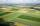I have a garden in the shape of a square with a side length of 0.02 kilometers. Neighbor has a large garden as well and one side of the garden we share. I'd bought from a neighbor garden that my area of my garden increased to 5 ares. a/ By how many acres
• Map - climb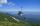On the map of High Tatras in scale 1:11000 are cable car stations in the Tatranska Lomnica and in the Skalnate Pleso with distance 354.6 mm. Altitude of this stations are 949 m and 1760 m. What is average angle of climb of this cable car track?
• RainfallA rectangular garden of 25m in length and width 20m in width fall 4mm of water. Express by a fraction in basic form what part of the 60-hectolitre tank we would fill with this water.
• Trapezoid RTThe plot has a shape of a rectangular trapezium ABCD, where ABIICD with a right angle at the vertex B. side AB has a length 36 m. The lengths of the sides AB and BC are in the ratio 12:7. Lengths of the sides AB and CD are a ratio 3:2. Calculate consumpti
• On the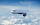On the map of Europe made at a scale of 1: 4000000, the distance between Bratislava and Paris is 28 cm. At what time an airplane flying at 800 km/h will fly this journey?
• Map scaleGarden has on plan on a scale of 1: 150 width 22 cm and length 35 cm. What is the real area of the garden?
• Bathroom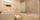How many CZK we pay for lining the perimeter walls of the bathroom with rectangular shape with dimensions 3.5 m and 4 m, high 1.5 m if 1 square m tile cost 300 CZK?
• Engine pulley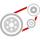The engine has a 1460 rev / min (RPM). Disc diameter is 350 mm. What will be the disc peripheral speed in RPM? Pulleys on the engine has diameter 80mm, on a disc has diameter 160mm.